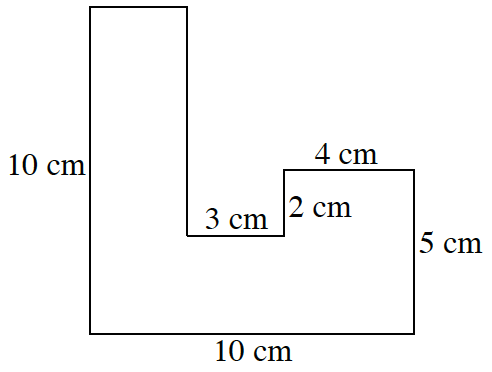### Home > CC1MN > Chapter 2 Unit 2 > Lesson CC1: 2.2.3 > Problem2-46

2-46.

Find the area and perimeter of the figure at right. All angles are right angles. Show all of your work.First, remember that the perimeter of a figure is the sum of the lengths of all the sides.

To find the perimeter, it would be helpful to find the unknown lengths of the sides (represented as a and b at right).

The top edge is  the bottom length 10 cm minus the other horizontal lengths (3 cm and 4 cm).

To find the unknown vertical side, look at all of the vertical lengths, 10 cm, 5 cm, and 2 cm. How do these lengths help you find the unknown side?

One way to find the area is to divide the figure into small squares of the same size. Here, you should use squares with sides of one centimeter like in the figure on the right. Now you can count the squares to find the area.

Using the formula Area = (length)(width), you can also easily find the area. First, divide the figure into separate rectangles and then find the individual areas with the formula mentioned before.

Don't forget to add all of the areas together!